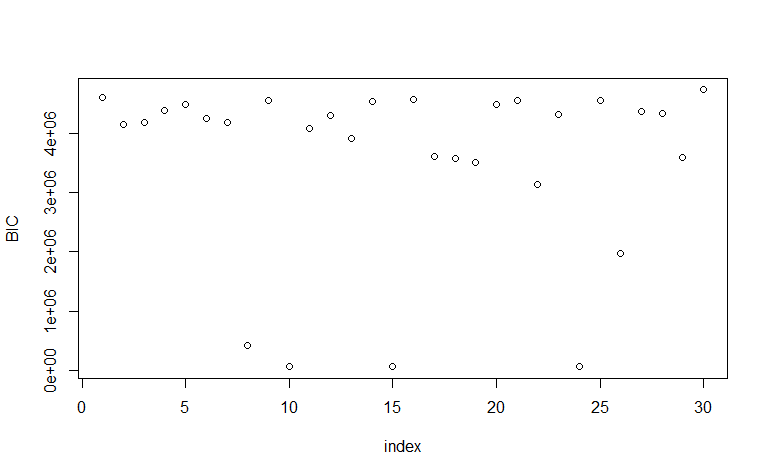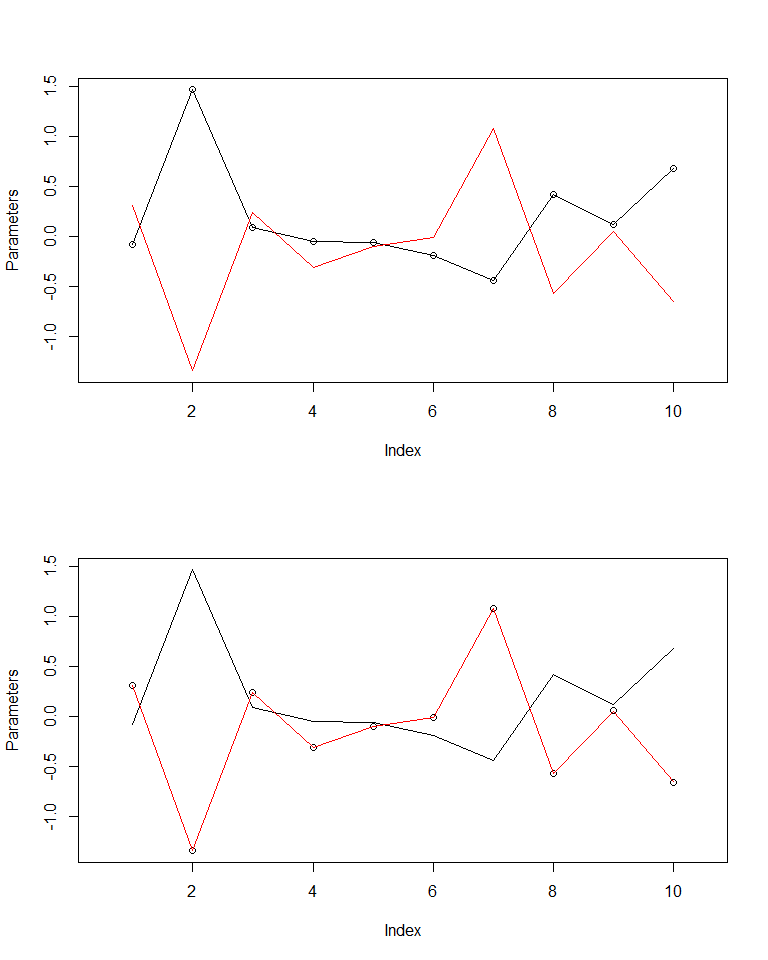# Warm-up and exposure in the EM algorithm

#### 2019-07-01

If you are using the EM algorithm, the chances are your data is quite complex - particularly you might have a large number of variables to consider. The issue with having a wide parameter space is that the EM algorithm suffers from getting caught in local minima. Here we detail how to avoid getting caught in such scenarios.

For this example we use a simulated dataset, ‘sim.3’, which is generated from two competing parameter spaces, and consists of 10 covariates and 1 output, with the added challenge of having an ‘exposure’.

The ‘small.em’ command has three key parameters in addition to the em.glm command: * ‘sample.size’ - set the number of observations to sample from ‘x’, ‘y’, and ‘weights’. * ‘repeats’ - set the number of random starts to perform. * ‘maxiter’ - set the maximum number of iterations to perform on each repeat.

Increasing either of these arguments will increase the time required to warm up the algorithm, but gives a better chance of finding the optimal state - we leave it to the reader to find their own heuristics.

In practice - the general approach is:

library(emax.glm)

warm_up <- small.em(
x = sim.3$x, y = sim.3$y,
K = 2, b.init = "random",
weight = sim.3$exposure, sample.size = 1000, repeats = 30, maxiter = 5 ) df <- summary(warm_up) kable(head(df)) index logLike bic 1 -2304008 4608044 2 -2077271 4154570 3 -2092235 4184498 4 -2195087 4390202 5 -2247419 4494866 6 -2124773 4249573 to produce multiple trials. We can see how BIC varies with the trial by plotting: plot(df$index, df$bic, xlab = "index", ylab = "BIC")For quality of life, we use ‘select_best’ to choose the optimal starting condition and can then pass this to the ‘em.glm’ algorithm to continue the optimization.  params <- select_best(warm_up) em <- em.glm( x = sim.3$x, y = sim.3$y, K = 2, b.init = params, weight = sim.3$exposure
)

and compare predicted (lines) and known (scatter) values:Fitted Parameters and known values

Resulting in reclaiming the original values.